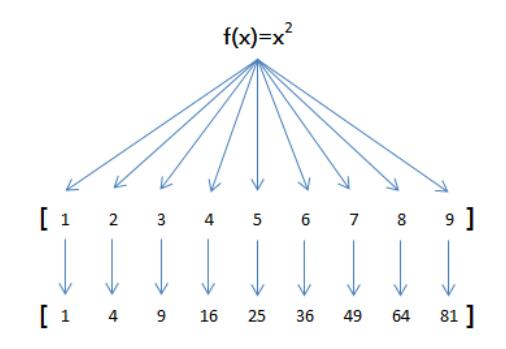# JavaScript之map reduce_动力节点Java学院整理

map``````
function pow(x) {
return x * x;
}

var arr = [1, 2, 3, 4, 5, 6, 7, 8, 9];
arr.map(pow); // [1, 4, 9, 16, 25, 36, 49, 64, 81]

``````

map()传入的参数是pow，即函数对象本身。 你可能会想，不需要map()，写一个循环，也可以计算出结果：

``````
var f = function (x) {
return x * x;
};

var arr = [1, 2, 3, 4, 5, 6, 7, 8, 9];
var result = [];
for (var i=0; i<arr.length; i++) {
result.push(f(arr[i]));
}

``````

``````
var arr = [1, 2, 3, 4, 5, 6, 7, 8, 9];
arr.map(String); // ['1', '2', '3', '4', '5', '6', '7', '8', '9']
``````

reduce

``````
[x1, x2, x3, x4].reduce(f) = f(f(f(x1, x2), x3), x4)
``````

``````
var arr = [1, 3, 5, 7, 9];
arr.reduce(function (x, y) {
return x + y;
}); // 25``````